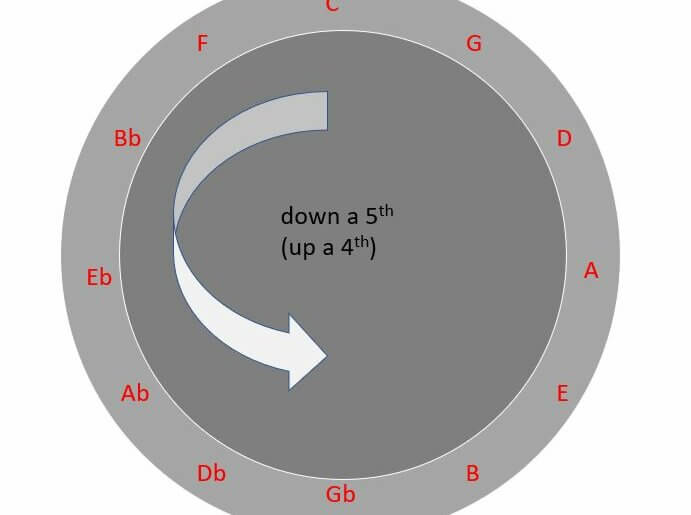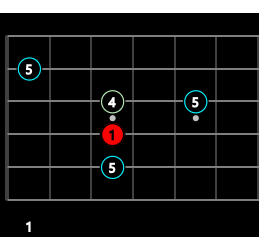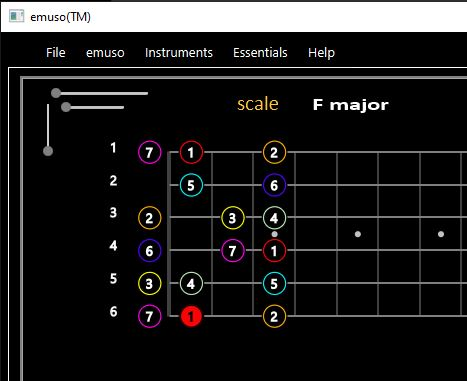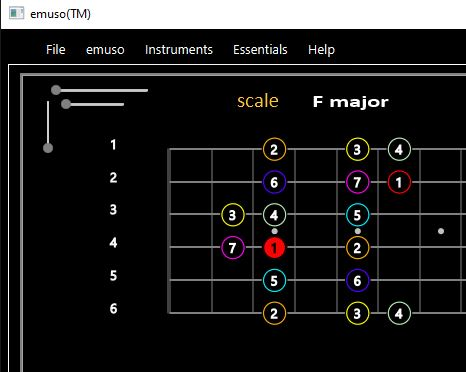# use the circle of 5ths to find IV and V of keyJerry

The circle of fifth has many uses.  Here is a handy tip, easy to use, it to find (remember) the root of the IV chord or V chord in any key.

## Method

Step 1:  choose the key of interest.  For example, the key of F.

Step 2: look clockwise on the circle to its neighbour.   This is the root note of the V chord of the key chosen in step 1.   If you chose F in step 1, then C is the root of the  V chord of F.

Step 3: look counter clockwise from the key chosen in step 1 to its neighbour.  This is the root note of the IV chord of the key chosen in step 1.  In you chose F in step 1, then Bb is the root of the  IV chord of F.

## Using guitar to find the IV and V chord roots in any key

This is really easy,if you know the interval shapes for the perfect fourth (4) and perfect fifth (5).

For example, the next diagram shows F (red circle) at fret 3 on the D string (4th sring)..   The treble E string (1st string) is the top horizontal line.  The bass E string (6th) is at the bottom horizontal line.   The “1” at the bottom left of the diagram is a fret number.

On the G (3rd) and B (2nd) strings, are shown the locations of notes that make a perfect 4th (labelled 4) or perfect 5th (labelled 5) with F.   The perfect 4th note is Bb.  The perfect 5th note is C.

The diagram also the correspond C an octave lower, at the 3rd fret on the A string (5th string).  So we label it the same, with a 5 (just imagine that the lower octave of F is also present, at the 1st fret on the bass E string.  The C on the A string makes a perfect 5th with that imagined F).## Interval inversions

What we’re about discuss would not be thought about at all when playing, but you should be aware of this.  Remember an interval always has just two pitches, never more or less

If the higher pitched note of an interval is dropped an octave, or the lower pitched note is raised an octave, we say the interval has been inverted.  Look at the above diagram, and the 5 (C) above the F.  Dropping that C an octave places it on the 3rd fret  on the A string.  When an interval is inverted, the number of semitones always changes.

If the interval is N semitones, then the inverted interval is always 12-N semitones (demonstrated below).

This means that a perfect 5th (7 semitones), inverts to an interval that is 12-7 = 5 semitones.  A perfect 4th is the name for 5 semitones.  So the inversion  of a perfect 5th is a perfect 4th.

Is this important to know?    When playing, the answer is very often not.   If you know a scale shape, you’ll know where the various scale members are found, and it’s much more use to associate the interval names used in the scale with the notes you see (visualise) in the scale shape.  For example, here are a couple of adjacent scale shapes for F major.When playing, you may think “I’ll end on a 5 (perfect 5th) because I want a stable sound”, so any of the pitches labelled 5 will do … each represents the fifth member of the major scale, but in different octaves.

But, if you are just literally given two pitches, and no other information, then it does matter. if you are doing a theory test on intervals say.  Now, you must answer based on the semitonesd involved, so you answer perfect 5th and perfect 4th respectively F with C above, or F with C below it, as discussed above.  And it will very much matter when using your ears.  You will definitely hear different sound flavours.  However, if you train your ears to recognise pitches relative to the tonal centre (regardless of octaves), then this doesn’t matter.

## Guitar tuning and interval (inversions)If we literally count semitone distances from the red circle (the pitch F) to the pitch labelled 5 above it, we would get 7 semitones … assuming standard guitar tuning.  This is because the open 3rd string is tuned 5 semitones higher that the open 4th string.  This means that any pair of pitches located at the same (aribtrary)  fret on these two strings are 5 semitones apart.  In which case, to get 7 semitones, we just need to choose a pitch 2 semitones higher (towards the body) than that on the treble string.

So, the C at the 5th fret on the G string must be 7 semitones above the F at the 3rd fret on the D string.

Similarly, the open 4th string is tuned 5 semitones higher than the open 5th string.  So, from the C at the 3rd fret on the A string to the F at the 3rd fret on the D string must be 5 semitones.